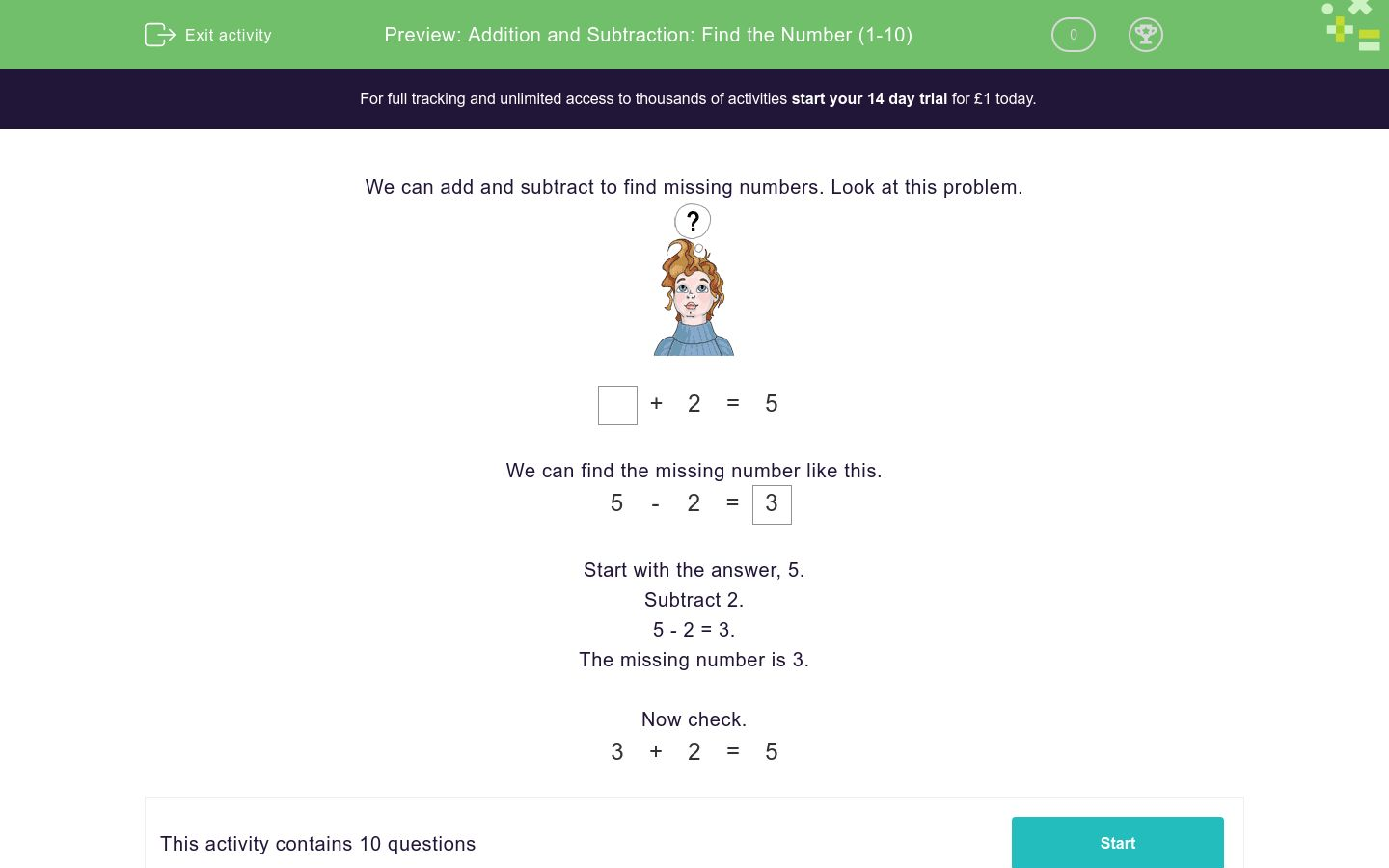# Addition and Subtraction: Find the Number (1-10)

In this worksheet, students find missing numbers using addition, subtraction and number bond knowledge.Key stage:  KS 1

Curriculum topic:   Number: Addition and Subtraction

Curriculum subtopic:   Solve Add/Subtract Problems to 20

Difficulty level:### QUESTION 1 of 10

We can add and subtract to find missing numbers. Look at this problem.+ 2 = 5

We can find the missing number like this.

 5 - 2 = 3

Subtract 2.

5 - 2 = 3.

The missing number is 3.

Now check.

 3 + 2 = 5

Find the missing number.? + 2 = 8

Find the missing number.? + 5 = 9

Find the missing number.? + 3 = 8

Find the missing number.? + 1 = 6

Find the missing number.? + 4 = 7

Find the missing number.? - 7 = 3

Find the missing number.? - 1 = 2

Find the missing number.? - 5 = 1

Find the missing number.? + 5 = 9

Find the missing number.? + 2 = 5

• Question 1

Find the missing number.? + 2 = 8

6
EDDIE SAYS
8 − 2 = 6
6 + 2 = 8
• Question 2

Find the missing number.? + 5 = 9

4
EDDIE SAYS
9 − 5 = 4
4 + 5 = 9
• Question 3

Find the missing number.? + 3 = 8

5
EDDIE SAYS
8 − 3 = 5
5 + 3 = 8
• Question 4

Find the missing number.? + 1 = 6

5
EDDIE SAYS
6 − 1 = 5
5 + 1 = 6
• Question 5

Find the missing number.? + 4 = 7

3
EDDIE SAYS
7 − 4 = 3
3 + 4 = 7
• Question 6

Find the missing number.? - 7 = 3

10
EDDIE SAYS
3 + 7 = 10
10 − 7 = 3
• Question 7

Find the missing number.? - 1 = 2

3
EDDIE SAYS
2 + 1 = 3
3 − 1 = 2
• Question 8

Find the missing number.? - 5 = 1

6
EDDIE SAYS
1 + 5 = 6
6 − 5 = 1
• Question 9

Find the missing number.? + 5 = 9

4
EDDIE SAYS
9 − 5 = 4
4 + 5 = 9
• Question 10

Find the missing number.? + 2 = 5

3
EDDIE SAYS
5 − 2 = 3
3 + 2 = 5
---- OR ----

Sign up for a £1 trial so you can track and measure your child's progress on this activity.

### What is EdPlace?

We're your National Curriculum aligned online education content provider helping each child succeed in English, maths and science from year 1 to GCSE. With an EdPlace account you’ll be able to track and measure progress, helping each child achieve their best. We build confidence and attainment by personalising each child’s learning at a level that suits them.

Get started